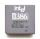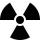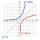# Sequence

Calculate what member of the sequence specified byhas value 86.

Result

n =  2

#### Solution:Leave us a comment of example and its solution (i.e. if it is still somewhat unclear...):Be the first to comment!## Next similar examples:

1. Geometric sequence 4It is given geometric sequence a3 = 7 and a12 = 3. Calculate s23 (= sum of the first 23 members of the sequence).
2. Geometric progression 2There is geometric sequence with a1=5.7 and quotient q=-2.5. Calculate a17.
3. Sequence - 5 membersWrite first five members of the sequence ?
4. Six termsFind the first six terms of the sequence a1 = -3, an = 2 * an-1
5. Computer revolutionWhen we started playing with computers, the first processor, which I remember was the Intel 8080 from 1974, with the performance of 0.5 MIPS. Calculate how much percent a year rose CPU performance when Intel 486DX from 1992 has 54 MIPS. What
6. BankPaul put 10000 in the bank for 6 years. Calculate how much you will have in the bank if he not pick earned interest or change deposit conditions. The annual interest rate is 3.5%, and the tax on interest is 10%.After 548 hours decreases the activity of a radioactive substance to 1/9 of the initial value. What is the half-life of the substance?
8. Logif ?, what is b?
9. DemographicsThe population grew in the city in 10 years from 42000 to 54500. What is the average annual percentage increase of population?
10. PopulationWhat is the population of the city with 3% annual growth, if in 10 years the city will have 60,000 residents?
11. Theorem proveWe want to prove the sentence: If the natural number n is divisible by six, then n is divisible by three. From what assumption we started?
12. Geometric progressionIn geometric progression, a1 = 7, q = 5. Find the condition for n to sum first n members is: sn≤217.
13. Exponential equationIn the set R solve the equation: ?
14. The city 3The city has 22,000 residents. How long it is expected to have 25,000 residents if the average annual population growth is 1.4%?
15. LogarithmDetermine the number whose decimal logarithm is -3.8.
16. CoordinateDetermine missing coordinate of the point M [x, 120] of the graph of the function f bv rule: y = 5x
17. Insert into GPBetween numbers 5 and 640 insert as many numbers to form geometric progression so sum of the numbers you entered will be 630. How many numbers you must insert?